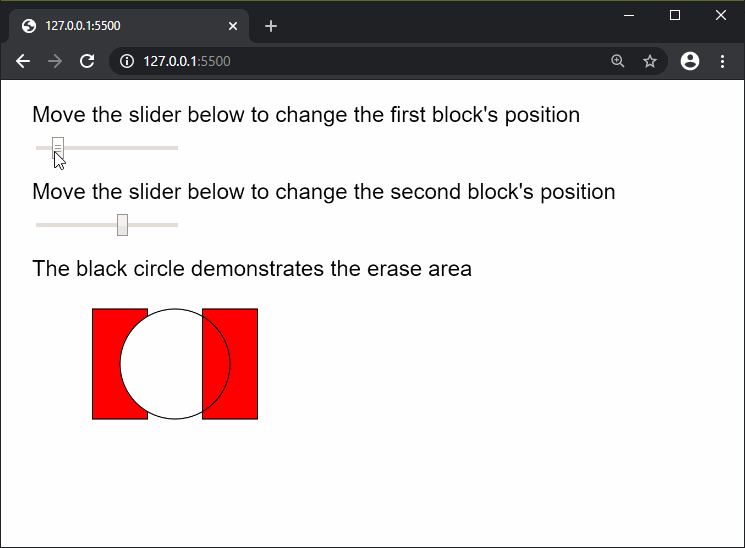# p5.js | noErase() Function

• Last Updated : 24 Apr, 2020

The noErase() function in p5.js is used to cancel the effects of the erase() function. It will return the functionality of the fill(), stroke(), and blendMode() functions to what they were before using erase(). Any drawing done after this function would be drawn normally.

Syntax:

`noErase()`

Parameters: This function does not accept any parameters.

Below example illustrates the noErase() function in p5.js:

Example:

 `function` `setup() {``    ``createCanvas(600, 400);``    ``textSize(20);``    ``firstBlockSlider = createSlider(50, 250, 75, 1);``    ``firstBlockSlider.position(30, 50);``  ` `    ``secondBlockSlider = createSlider(50, 250, 175, 1);``    ``secondBlockSlider.position(30, 120);``}``  ` `function` `draw() {``    ``clear();``    ``fill(``'black'``);``    ``text(``"Move the slider below to change the"``        ``+ ``" first block's position"``, 20, 30);``    ``text(``"Move the slider below to change the"``        ``+ ``" second block's position"``, 20, 100);``    ``text(``"The black circle demonstrates the"``        ``+ ``" erase area"``, 20, 170);``  ` `    ``fill(``'red'``);``    ``rect(firstBlockSlider.value(), 200, 50, 100);``  ` `    ``// Start erasing with erase()``    ``erase();``    ``circle(150, 250, 100);``  ` `    ``// Stop erasing with noErase()``    ``noErase();``  ` `    ``fill(``'red'``);``    ``rect(secondBlockSlider.value(), 200, 50, 100);``  ` `    ``// Circle to illustrate the erase position``    ``noFill();``    ``circle(150, 250, 100);``}`

Output:Reference: https://p5js.org/reference/#/p5/noErase

My Personal Notes arrow_drop_up